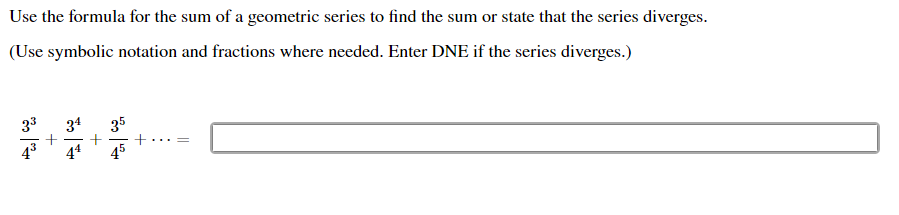# Use the formula for the sum of a geometric series to find the sum or state that the series diverges. (Use symbolic notation and fractions where needed. Enter DNE if the series diverges.) 43 44

Questionhelp_outlineImage TranscriptioncloseUse the formula for the sum of a geometric series to find the sum or state that the series diverges. (Use symbolic notation and fractions where needed. Enter DNE if the series diverges.) 43 44 fullscreen